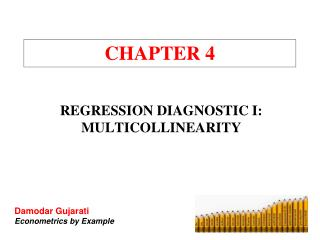# CHAPTER 4 - PowerPoint PPT PresentationDownload PresentationCHAPTER 4

CHAPTER 4
Download Presentation## CHAPTER 4

- - - - - - - - - - - - - - - - - - - - - - - - - - - E N D - - - - - - - - - - - - - - - - - - - - - - - - - - -
##### Presentation Transcript

1. CHAPTER 4 REGRESSION DIAGNOSTIC I: MULTICOLLINEARITY Damodar Gujarati Econometrics by Example

2. MULTICOLLINEARITY • One of the assumptions of the classical linear regression (CLRM) is that there is no exact linear relationship among the regressors. • If there are one or more such relationships among the regressors, we call it multicollinearity, or collinearity for short. • Perfect collinearity: A perfect linear relationship between the two variables exists. • Imperfect collinearity: The regressors are highly (but not perfectly) collinear. Damodar Gujarati Econometrics by Example

3. CONSEQUENCES • If collinearity is not perfect, but high, several consequences ensue: • The OLS estimators are still BLUE, but one or more regression coefficients have large standard errors relative to the values of the coefficients, thereby making the t ratios small. • Even though some regression coefficients are statistically insignificant, the R2 value may be very high. • Therefore, one may conclude (misleadingly) that the true values of these coefficients are not different from zero. • Also, the regression coefficients may be very sensitive to small changes in the data, especially if the sample is relatively small. Damodar Gujarati Econometrics by Example

4. VARIANCE INFLATION FACTOR • For the following regression model: • It can be shown that: • and • where σ2 is the variance of the error term ui, and r23 is the coefficient of correlation between X2 and X3. Damodar Gujarati Econometrics by Example

5. VARIANCE INFLATION FACTOR (CONT.) • is the variance-inflating factor. • VIF is a measure of the degree to which the variance of the OLS estimator is inflated because of collinearity. Damodar Gujarati Econometrics by Example

6. DETECTION OF MULTICOLLINEARITY • 1. High R2 but few significant t ratios • 2. High pair-wise correlations among explanatory variables or regressors • 3. High partial correlation coefficients • 4. Significant F test for auxiliary regressions (regressions of each regressor on the remaining regressors) • 5. High Variance Inflation Factor (VIF) and low Tolerance Factor (TOL, the inverse of VIF) Damodar Gujarati Econometrics by Example

7. REMEDIAL MEASURES • What should we do if we detect multicollinearity? • Nothing, for we often have no control over the data. • Redefine the model by excluding variables may attenuate the problem, provided we do not omit relevant variables. • Principal components analysis: Construct artificial variables from the regressors such that they are orthogonal to one another. • These principal components become the regressors in the model. • Yet the interpretation of the coefficients on the principal components is not as straightforward. Damodar Gujarati Econometrics by Example